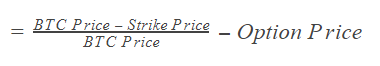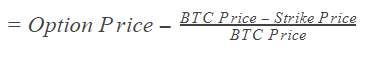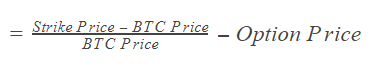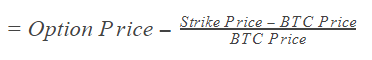In this lesson we’ll be working through some practical examples of how to calculate the profit and loss of option positions on Deribit. We will be using BTC in the examples to keep things as simple as possible, but the same calculations hold true for ETH options as well. We will ignore fees to keep the examples as simple as possible. To adjust for fees though, you would subtract the fees from the final profit/loss figure.

The profit/loss calculations for options might at first seem a little more complicated than the ones for futures. However, just like any trade, no matter which direction you play it in you will have a buy order and a sell order. To calculate the profit you need to calculate the difference between these two points. General formulas are included at the end.

## Closing an option trade early

While the options on Deribit are all European, and therefore cannot be exercised early, you are still free to close an option position at any time by reducing your position to zero. When an option position is not held to expiry, but closed early, the profit and loss calculation is very simple. Every trade involves a buy order and a sell order, so you simply subtract what you bought the option for from what you sold it for.

Example 1:
You sell a bitcoin call option and collect a premium of 0.15 BTC. Several weeks later the option has lost some value and currently has a best ask of 0.05 BTC. You decide to close this position by executing a buy order at the available price of 0.05 BTC.

Your profit/loss is what you sold the option for minus what you bought the option for. Your sell price was 0.15 BTC, and your buy price was 0.05 BTC, so:
0.15 – 0.05 = 0.1 BTC

Example 2:
You buy a bitcoin call option and pay a premium of 0.1 BTC. A few days later the option has gained some value and has a current best bid of 0.18 BTC. You decide to close this position by executing a sell order at the available price of 0.18 BTC.

The calculation is the same as before. Your sell price was 0.18 BTC, and your buy price was 0.1 BTC, so:
0.18 – 0.1 = 0.08 BTC

Example 3:
You buy a bitcoin put option and pay a premium of 0.12 BTC. Some time later the option has lost some value and has a current best bid of 0.1 BTC. You decide to close this position by executing a sell order at the available price of 0.1 BTC.

0.1 – 0.12 = -0.02 BTC

Notice that we didn’t need any of the details of the option or current price of bitcoin to calculate the profit/loss for each of these first 3 positions. As you’ve closed the position early, all that matters is the price of your opening and closing orders.

Before an option expires, the price of the option at any given time will depend on several factors including volatility, time to expiry and the underlying price. To calculate the profit/loss though, you don’t need to take account of any of this, it’s simply what you sold it for minus what you bought it for.

## Options held to expiry

At expiration, all of the extrinsic value resulting from volatility and time is gone. To calculate the value of an option when held to expiry we just need to know the strike price and the delivery price at expiration.

Any value the option has at expiry is transferred from the seller to the buyer. To calculate the total profit/loss all that then needs to be done is to adjust for the initial premium paid.

Note: The delivery price, i.e. the price which is used to calculate an option’s value when it expires, is calculated as a 30 minute TWAP (time weighted average price) of the Deribit Bitcoin index.

Example 4 – ITM long call option:
You buy a bitcoin call option with a strike price of \$10,000 and pay a price of 0.05 BTC. You hold this option until it expires. At expiration, the price of bitcoin is \$12,500.

The value of this call option is calculated as the difference between the delivery price of \$12,500, and the strike price of \$10,000.

\$12,500 – \$10,000 = \$2,500

So the option has a value of \$2,500. To calculate how much this is in bitcoin, you divide this value by the current price of \$12,500.

\$2,500 / \$12,500 = 0.2 BTC

This 0.2 BTC is paid from the seller to you, the buyer. To calculate your final profit you subtract from this number the original premium you paid for the option, which was 0.05 BTC.

0.2 – 0.05 = 0.15 BTC

Note here that when holding to expiry, you only executed one side of the trade manually (a buy order in this case). When the option expires the delivery process closes this position off for you automatically, effectively creating the sell side of your trade.

Example 5 – ITM long call option:
You buy a bitcoin call option with a strike price of \$8,000 and pay a price of 0.25 BTC. You hold this option until it expires. At expiration, the price of bitcoin is \$10,000.

The value of this call option can be calculated as:

\$10,000 – \$8,000 = \$2,000

To calculate how much this is in bitcoin, you divide by the current price of \$10,000 to give:

\$2,000 / \$10,000 = 0.2 BTC

This 0.2 BTC is paid from the seller to you. Your final profit can be calculated as:

0.2 BTC – 0.25 BTC = -0.05 BTC

Notice how just as with example 4, this call option finished in the money, and therefore had some value at expiry. However, this time the eventual value of the option was not enough to make up for the price paid for the option, therefore the position resulted in a small loss.

Example 6 – ITM long put option:
You buy a bitcoin put option with a strike price of \$10,000 and pay a price of 0.16 BTC. You hold this option until it expires. At expiration, the price of bitcoin is \$5,000.

The value of this put option can be calculated as:

\$10,000 – \$5,000 = \$5,000

To calculate how much this is in bitcoin, you divide by the current price of \$5,000 to give:

\$5,000 / \$5,000 = 1 BTC

This 1 BTC is paid from the seller to you. Your final profit can be calculated as:

1 BTC – 0.16 BTC = 0.84 BTC

Example 7 – ITM long put option:
You buy a bitcoin put option with a strike price of \$9,000 and pay a price of 0.2 BTC. You hold this option until it expires. At expiration, the price of bitcoin is \$8,000.

The value of this put option can be calculated as:

\$9,000 – \$8,000 = \$1,000

To calculate how much this is in bitcoin, you divide by the current price of \$8,000 to give:

\$1,000 / \$8,000 = 0.125 BTC

This 0.125 BTC is paid from the seller to you. Your final profit can be calculated as:

0.125 BTC – 0.2 BTC = -0.075 BTC

As with the call option in example 5, the price moved in the correct direction for your position and this put option finished in the money. However the bitcoin price did not move far enough to make up for the price paid for the option, therefore the position made a loss.

## Options that expire out of the money

In examples 4 to 7, we only mentioned options that expired in the money. This is because when an option expires out of the money, it has no value therefore there is nothing to be transferred from the seller to the buyer. In this case the buyer will have a loss equal to the premium paid, and the seller will have a profit equal to the premium paid.

## Profit/loss when selling options

The previous examples are all from the buyers point of view. The calculation for the seller’s profit/loss is simply the negative of the buyer’s.

Example 8 – ITM short call option:
You sell a bitcoin call option with a strike price of \$12,000 and collect a premium of 0.09 BTC. You hold the position until it expires. At expiration, the price of bitcoin is \$15,000.

The value of this call option can be calculated as:

\$15,000 – \$12,000 = \$3,000

To calculate how much this is in bitcoin, you divide by the current price of \$15,000 to give:

\$3,000 / \$15,000 = 0.2 BTC

As in this instance you are the seller, this 0.2 BTC is paid from your account to the buyer. To calculate your final profit/loss you subtract this number from the premium you originally collected, which was 0.09 BTC.

0.09 BTC – 0.2 BTC = -0.11 BTC

Example 9 – ITM short call option:
You sell a bitcoin call option with a strike price of \$9,000 and collect a premium of 0.18 BTC. You hold the position until it expires. At expiration, the price of bitcoin is \$10,000.

The value of this call option can be calculated as:

\$10,000 – \$9,000 = \$1,000

To calculate how much this is in bitcoin, you divide by the current price of \$10,000 to give:

\$1,000 / \$10,000 = 0.1 BTC

As you are the seller, this 0.1 BTC is paid from your account to the buyer. To calculate your final profit/loss you subtract this number from the premium you originally collected, which was 0.18 BTC.

0.18 BTC – 0.1 BTC = 0.08 BTC

Example 10 – ITM short put option:
You sell a bitcoin put option with a strike price \$12,000 and collect a premium of 0.3 BTC.
You hold the position until it expires. At expiration, the price of bitcoin is \$8,000

The value of this put option can be calculated as:

\$12,000 – \$8,000 = \$4,000

To calculate how much this is in bitcoin, you divide by the current price of \$8,000 to give:

\$4,000 / \$8,000 = 0.5 BTC

This 0.5 BTC is paid from your account to the buyer. To calculate your final profit/loss you subtract this number from the premium you originally collected, which was 0.3 BTC.

0.3 BTC – 0.5 BTC = -0.2 BTC

## General formulas

For in the money call options at expiry:
The call buyer’s profit/loss in BTC is calculated as:The call seller’s profit/loss of course is the opposite, and can be calculated as:For in the money put options at expiry:
The put buyer’s profit/loss in BTC is calculated as:The put seller’s profit/loss of course is the opposite, and can be calculated as:You should now be able to calculate profit/loss for any option on Deribit, for both buyer and seller. For positions with multiple legs, the total PNL is equal to the sum of the PNL of each of the options contained within it.

For all of the examples in this lesson, all figures are given per 1 contract. For different sized positions you simply multiply the PNL by the number of contracts you have. For example if you have a position size of 0.4 contracts, multiply the PNL result by 0.4. If you have a position size of 25 contracts, multiply the PNL result by 25.

You can visualise the PNL of both option and futures positions with our Position builder. This can be found at: pb.deribit.com
And there is a tutorial to get you started using it here.

This is a powerful tool that allows you to generate notional portfolios and evaluate their PNL potential. It also includes the ability to import your current Deribit account portfolio.

AUTHOR(S)CryptarbitrageMrJozza